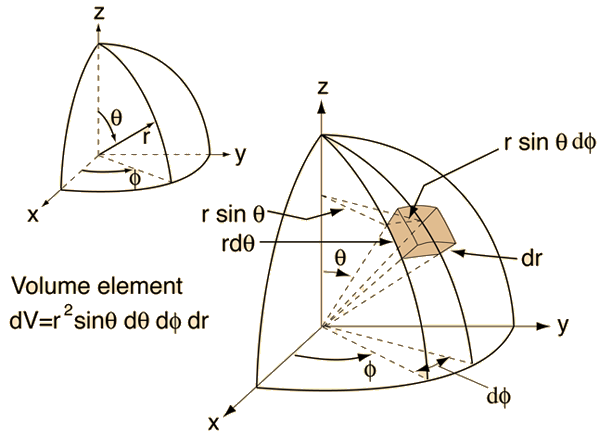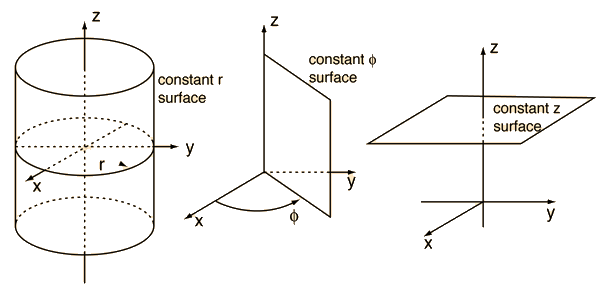# Spherical Polar CoordinatesIndex

 HyperPhysics****HyperMath*****Geometry R Nave
Go Back

# Cylindrical Polar CoordinatesWith the axis of the circular cylinder taken as the z-axis, the perpendicular distance from the cylinder axis is designated by r and the azimuthal angle taken to be Φ.Index

 HyperPhysics****HyperMath*****Geometry R Nave
Go Back

# Applications of Spherical Polar Coordinates

Physical systems which have spherical symmetry are often most conveniently treated by using spherical polar coordinates.

 Hydrogen Schrodinger Equation Maxwell speed distribution Electric potential of sphere
Index

 HyperPhysics****HyperMath*****Geometry R Nave
Go Back

# Applications of Cylindrical Polar Coordinates

Physical systems which have cylindrical symmetry are often most conveniently treated by using cylindrical polar coordinates.

 Cylindrical capacitor Electric field of line charge.
Index

 HyperPhysics****HyperMath*****Geometry R Nave
Go Back